## Friday, February 17, 2006

### Cyclotomic Integers

In Ernst Kummer's groundbreaking partial proof on Fermat's Last Theorem, he used cyclotomic integers.

Cyclotomic integers are based the equation xn = 1 (they get their name from this equation which is known as the cyclotomic equation). The solutions to this equation are known as the Roots of Unity. The solutions to n=1 and n=2 are well known (1, -1). What is less well known is that as n increases, there are complex solutions that also solve this equation (these are also known as DeMoivre Numbers).

For some, it may seem strange that complex numbers emerge out of x3 = 1. The insight to why this is so comes from considering Euler's Identity:

e=-1

In my next blog, I will go into the details behind this equation. Still, using this equation, we can see that three solutions to x3 = 1 are:
1, e(2iπ)/3, e(4iπ)/3

Since (e(2iπ)/3)3 = e2iπ = (-1)2 = 1.

The solution then for any value of n is e(2iπ)/n and this solution is by convention represented by the Greek symbol zeta ζ. The set of integers then is Z[ζ] where each integer can be constructed from the rational integers a,b using a + bζ. This construction follows the same pattern as what Euler did for Z[√-3] (see here) and what Gauss did for Z[i] (see here).

For any value n, there are n different roots of unity which can be generated by e(2iπk)/n where k is any integer 0, 1, 2, ..., up to n-1. For this reason, one speaks about the value ζ (equal to e2iπ/n) as a primitive root of unity.

One might reasonably ask if all cyclotomic integers created in this way are characterized by unique factorization. In using quadratic integers, Euler made a mistake by assuming that Z[√-3] has unique factorization which it does not. Gabriel Lame assumed that all cyclotomic integers where characterized by unique factorization when he presented his proof of Fermat's Last Theorem. This assumption turns out to be the major flaw in Lame's proof.

This then becomes the major question which Kummer sought to resolve. Under what circumstances are cyclotomic integers characterized by unique factorization? The answer to this question led Kummer to his important partial proof of Fermat's Last Theorem. He showed that Fermat's Last Theorem holds for a certain set or primes which he called "regular primes." I talk more about the properties of cyclotomic integers here.

References

## Wednesday, February 15, 2006

In today's blog I continue the proof for the Principle of Quadratic Reciprocity. If you are not familiar with the concepts of quadratic reciprocity, quadratic residues, or the Legendre Symbol start here.

If you would like to start this proof at the beginning, start here.

Today's proof is taken from Gareth A. Jones and J. Mary Jones' Elementary Number Theory.

Lemma 1:
(a) Let x' = (p+1)/2 - x
(b) Let y' = (q+1)/2 - y
Then
qx - py is greater than -(p/2) if and only if qx' - py' is less than q/2.

(1) Assume that qx - py is greater than (-p/2)

(2) (p/2) + qx - py is greater than 0

(3) (p +q-q+pq-pq)/2 + qx - py is greater than 0

(4) (p - q + pq - pq)/2 + qx - py is greater than (-q/2)

(5) -q(p+1)/2 + p(q+1)/2 + qx - py is greater than (-q/2)

(6) q(p+1)/2 - p(q+1)/2 - qx + py is less than (q/2)

(7) q[(p+1)/2 - x] - p[(q+1)/2 - y] is less than (q/2)

(8) qx' - py' is less than (q/2)

(9) We can use the same reasoning to show that:
if qx' - py is less than (q/2), then qx - py is greater than (-p)/2.

QED

Lemma 2: Given:
(a) Let P = { 1, 2, ... (p-1)/2 }
(b) Let Q = {1, 2, ... (q-1)/2 }
(c) Let A be the set of points PxQ such that qx-py is greater than (-p)/2. [See diagram in Lemma 3]
(d) Let B be the set of points PxQ such that qx-py is less than (q/2) [See diagram in Lemma 3]
(e) Let α be the number of points in A
(f) Let β be the number of points in B
Then:
There is a one-to-one mapping between α and β [So that, they both have the same number of points]

Proof:

(1) Let's define the following function:
p(x,y) = (x',y') = [ (p+1)/2 - x, (q+1)/2 - y ]

(2) p(A) = p(B) since qx - py is greater than (-p)/2 implies that qx' - py is less than (q/2). [See Lemma 1 above]

(3) p(B) = p(A) since qx - py is less than (q/2) implies that qx' - py' is greater than (-p)/2. [See Lemma 1 above]

QED

Lemma 3: Given
(a) p,q are distinct, odd primes
(b) P is a set = { 1, 2, ... (p-1)/2 }
(c) Q is a set = { 1, 2, ... (q-1)/2 }
(d) x,y are integers such that x ∈ P and y ∈ Q
(e) (-p/2) is less than qx - py which is less than (q/2).
(f) μ = the number of elements (x,y) ∈ P x Q where (-p/2) is less than qx - py which is less than -1.
(g) ν = the number of elements (y,x) ∈ Q x P where (-q/2) is less than py - qx which is less than -1.

Then:
(-1)μ + ν = (-1)[(p-1)(q-1)]/4

Proof:

(1) The set of assumptions can be represented as a graph:We see that:
(a) R is the set of all points PxQ = [(p-1)/2][(q-1)/2] = (p-1)(q-1)/4

(b) μ + ν = R ∩ S

(c) A = the area of PxQ that falls above S

(d) B = the area of PxQ that falls below S

(2) We can further define α, β such that:

α = the number of points ∈ A
β = the number of points ∈ B

(3) From this, we see that the number of points R ∩ S is:
(p-1)(q-1)/4 - (α + β)

(4) Now we can show that α = β or in other words that there is a one-to-one mapping between elements in α with elements in β by the following:

(a) We can do this using the following rotation p(x,y):

p(x,y) = (x',y') = ( [p+1]/2 - x, [q+1]/2 - y )

(b) So, for example, the corners of R before the rotation are: (1,[q-1]/2), ([p-1]/2,[q-1]/2), (1,1), and ([p-1]/2, 1).

(c) After the rotation, the corners of R' are: ([p-1]/2,1), (1,1), ([p-1]/2,[q-1]/2), and (1,[q-1]/2).

(d) So, we see that R' has the same corners as R.

(e) By Lemma 2, we see that there is a one-to-one correspondence between A and B.

(5) So, this means that α + β ≡ 2*α ≡ 0 (mod 2).

(6) But this means that the α + β does not change the result since:
(-1)μ + ν = (-1)μ + ν*(-1)

So:
(-1)μ + ν = (-1)μ + ν + 2*α = (-1)(p-1)(q-1)/4

QED

## Monday, February 13, 2006

### Quadratic Reciprocity: Expanding on Gauss's Lemma

In today's blog I continue the proof for the Principle of Quadratic Reciprocity. If you are not familiar with the concepts of quadratic reciprocity, quadratic residues, or the Legendre Symbol start here.

If you would like to start this proof at the beginning, start here.

Today's proof is taken from Gareth A. Jones and J. Mary Jones' Elementary Number Theory.

Lemma 1:
if
(a) p,q are odd primes
(b) P = the set of integers {1, 2, ..., (p-1)/2}
(c) N = the set of integers P = { -1, -2, ..., -(p-1)/2 }
(d) qx ≡ n (mod p) where n ∈ N, x ∈ P
then:
there exists a y such that (-p/2) is less than (qx - py) which is less than 0.

Proof:

(1) There exists y such that qx - n = py [Since qx ≡ n (mod p) -- see here for review of modular arithmetic]

(2) This then gives us: qx - py = n.

(3) qx - py is less than 0 since n is less than 0.

(4) qx - py is greater than (-p/2) since n ≥ -(p-1)/2 = (-p+1)/2 which is greater than -p/2.

QED

Lemma 2: if p,q,x,y are integers such that:
(a) p,q are positive, odd primes
(b) (-p/2) is less than qx - py
(c) qx - py is less than 0
(d) x,y are positive.
Then:
(a) there is only 1 value that y can have.
(b) 0 is less than qx/p which is less than y
(c) y is less than (qx/p) + 1/2.

Proof:

(1) Let y be any integer that satisfies the conditions (a),(b),(c)

(2) There is no value y' that y' is an integer and less than y which satisfies (a),(b),(c) since:

(a) qx - p(y-1) = qx - py + p

(b) We know that absolute(p) is greater than absolute(qx-py) since (-p/2) is less than (qx-py) which is less than 0.

(c) Since p is positive and qx-py is negative, we know that qx-py + p is greater than 0.

(3) There is no value y' such that y' is an integer and greater than y which satisfies (a),(b),(c) since:

(a) qx - p(y+1) = qx - py - p

(b) Since -p is greater than -p/2, we know that qx-py-p is less than (-p/2).

(4) From (a),(b),(c), we know that (p/2) is greater than (py-qx) which is greater than 0.

(5) Dividing (4) by p gives us:
(1/2) is greater than y - (qx/p) which is greater than 0.

(6) From (5), we know that y is greater than (qx/p).

(7) From (5), we also know that (1/2) + (qx/p) is greater than y.

(8) Since q,x,p are positive, we know that qx/p is greater than 0.

(9) By (#8),(#6), we know that y is greater than 0.

QED

Lemma 3: if q,p,x,y are integers such that:
(a) p,q are positive, odd primes
(b) (-p/2) is less than qx - py
(c) qx - py is less than 0
(d) x ∈ { 1, 2, ..., (p-1)/2 }
(e) y is a positive integer
Then:
y is less than (qx/p) + 1/2 ≤ [q(p-1)]/2p + 1/2 which is less than q+1/2

Proof:

(1) From Lemma 2, we know that:
0 is less than (qx/p) which is less than y which is less than (qx/p) + 1/2.

(2) From (d), we know that x ≤ (p-1)/2.

(3) Combining (1) and (2), we have:
(qx/p) + (1/2) ≤ [q(p-1)]/2p + (1/2)

(4) We can conclude that [q(p-1)]/2p + (1/2) is less than (q+1)/2 since:

(a) [q(p-1)]/2p + (1/2) = (qp - q + p)/2p = [(qp/p - q/p + p/p)]/2 =
= (q - q/p + 1)/2 = (q + 1 - q/p)/2

(b) q/p is greater than 0 since q,p are odd primes.

(c) From (a) and (b), we have:
(q + 1 - q/p)/2 is less than (q+1)/2 [ Since 1 - q/p is less than 1 ]

QED

Lemma 4: if q,p are odd primes, then there exist μ, ν where:

(a) Using the Legendre Symbol (see here):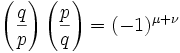(b) P = set of integer = {1, 2, ... (p-1)/2 }

(c) Q = set of integers = {1, 2, ... (q-1)/2 }

(d) μ + ν = the number of pairs (x,y) ∈ P x Q such that -p/2 is less than (qx-py) which is less than q/2.

Proof:

(1) We can start out with the observation that: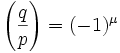where μ is the number of pairs (x,y) ∈ PxQ such that (-p/2) is less than qx-py which is less than 0 since:

(a) From Gauss's Lemma (see here), we have:where μ is the number if elements in qP ∩ N where N = of integers -P, that is, {-1, -2, ... -(p-1)/2}

(b) qP ∩ N → qx ≡ n (mod p) where n ∈ N, x ∈ P. [See Gauss's lemma for details if needed, see here for review of modular arithmetic]

(c) There exists y such that (-p/2) is less than qx - py which is less than 0. [By Lemma 1 above]

(d) We know that y is greater than 0 [See Lemma 2 above]

(e) Also, y is less than (q+1)/2. [See Lemma 3 above]

(f) So, y ≤ (q-1)/2 [ since there is no integer greater than (q-1)/2 and less than (q+1)/2 ]

(g) So, y ∈ Q since (d) gives us y ≥ 1 and (f) gives us y ≤ (q-1)/2.

(h) Since x ∈ P from assumption, we have (x,y) ∈ PxQ [see here if you need a review of PxQ notation]

(2) We can use the same reasoning as (#1) to get a value ν such that:where ν is the number of pairs (y,x) ∈ QxP such that (-q/2) is less than py-qx which is less than 0 .

(3) Combining (1) and (2) gives us:where if x,y are taken from (#1), we have:

(x,y) ∈ PxQ such that (-p/2) is less than qx-py which is less than 0

If x,y are taken from (#2), then we have:

(y,x) ∈ QxP such that (-q/2) is less than py-qx which is less than 0

(4) Combining both possibilities, we have:

(x,y) ∈ PxQ, (-p/2) is less than qx-py which is less than (q/2).

QED

## Sunday, February 12, 2006

### Euler's Criterion

In today's blog I continue the proof for the Principle of Quadratic Reciprocity. If you are not familiar with the concepts of quadratic reciprocity, quadratic residues, or the Legendre Symbol start here.

If you would like to start this proof at the beginning, start here.

If you are not familiar with the Legendre Symbol, you should review here.

Today's proof is taken from Gareth A. Jones and J. Mary Jones' Elementary Number Theory.

Definition 1: Qn: the set of quadratic residues

That is, these are the integers a mod n where a ≡ s2 (mod n) and s ∈ Un

NOTE: See here for a review of Un

Examples:

Q7 = { 1, 2, 4 }

12 ≡ 1 (mod 7) [ 1 ∈ U7 since 1 ≡ 1 (mod 7) ]
22 ≡ 4 (mod 7) [ 4 ∈ U7 since (4)(2) ≡ 1 (mod 7)]
42 ≡ 2 (mod 7) [ 2 ∈ U7 since (2)(4) ≡ 1 (mod 7)]

Q8 = { 1 }

12 ≡ 1 (mod 8)

Lemma 1: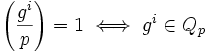Proof:

This is true by the the definition of the Legendre Symbol (see here)

QED

Lemma 2: if p is an odd prime and g is a primitive root for Up, then gi ∈ Qp if and only if i is even.

Proof:

(1) if i is even, then gi/2 ∈ Up [See here for definition of primitive root]

(2) But gi/2 ∈ Up, then gi ≡ (gi/2)2 (mod p) and therefore gi ∈ Qp [See above for definition of Qp]

(3) if gi ∈ Qp, then there exists j such that gi ≡ (gj)2 (mod p) [Based on definition of Qp]

(4) so, i ≡ 2j (mod φ(p)) since:

(a) φ(p) is the order of g. [See detail here]

(b) There exists q,r such that [from the Division Algorithm, see here]:
i = q(φ(p)) + r
0 ≤ r which is less than φ(p)

(c) And, i ≡ r (mod φ(p))

(d) So, gi ≡ gq(φ(p))+r ≡ (gφ(p))q*gr ≡ (1)q*gr ≡ gr (mod p)

(e) There exists, q', r' such that:
2j = q'(φ(p)) + r'
0 ≤ r' which is less than φ(p)

2j ≡ r' (mod φ(p))

(f) Now it follows that r = r' since:
g2j ≡ (1)q'*gr' ≡ gr' ≡ gr (mod p)

(g) Putting this all together, gives us:
2j ≡ i ≡ r (mod p)

(5) Now φ(p) is even. [since φ(p) = p-1 and p is an odd prime -- see here for details.]

(6) So i is even since:

(a) There exists a such that φ(p) = 2a [Since φ(p) is even]

(b) There exists b such that i - 2j = (2a)b [By definition of modular arithmetic, see here]

(c) So, 2 must divide i.

QED

Lemma 3: If p is an odd prime and g is a primitive root mod p, then: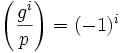Proof:

(1) Both values are either equal to 1 or -1. [See here for definition of Legendre Symbol]

(2) So, I will prove that:In other words, it is 1 only if i is even.

(3) In order for it to be 1, gi must be an element of Qp. [See Lemma 1 above]

(4) From Lemma 2, we know that gi ∈ Qp if and only if i is even.

QED

Theorem 1: Euler's Criterion: if p is an odd prime and a is any integer then: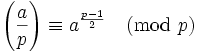Proof:

(1) We can assume that p does not divide a since if it did: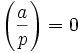and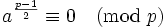which proves the conclusion.

(2) Now, if p doesn't divide a, then gcd(p,a)=1 since p is a prime.

(3) Also, there exists a' such that a ≡ a' (mod p) and a' is a unit mod p since:

(a) gcd(p,a)=1 → there exists u,v such that au + pv = 1 (By Bezout's Identity, see here for proof)

(b) So, au - 1 = pv → au ≡ 1 (mod p)

(c) But then a is a unit mod p (see here for definition of a unit mod p)

(4) The set of units mod p is cyclic (see here for proof)

(5) Then, there exists an element g such that g is the primitive root for the set of units mod p. (see here for definition of cyclic)

(6) So, there exists i such that a ≡ gi (mod p) (see here for definition of primitive root)

(7) Let h ≡ g(p-1)/2 (mod p)

(8) h2 ≡ gp-1 (mod p) [See here for a review of exponents if needed]

(9) φ(p) = p-1 [See here for details.]

(10) gp-1 ≡ gφ(p) ≡ 1 (mod p) [See Euler's Theorem]

(11) So p divides gp-1-1 → p divides h2 - 1 → p divides (h-1)(h+1)

(12) So, h ≡ 1 or h ≡ -1 (mod p)

(13) But h cannot be ≡ 1 (mod p) since (p-1)/2 is less than p-1 and p-1 is the order [See here for the definition of order]

(14) So h ≡ -1 (mod p)

(15) From (#6), we have: a(p-1)/2 ≡ (gi)(p-1)/2 ≡ (g(p-1)/2)i ≡ hi ≡ (-1)i (mod p)

(16) Now from Lemma 3 above:(17) Since a ≡ gi (mod p), we also have: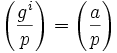(18) Finally, since a(p-1)/2 ≡ (-1)i (mod p) from#15, we have:QED